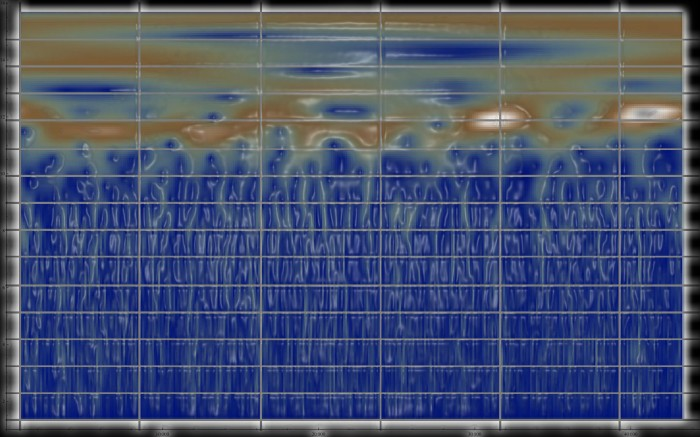# The Mathematica Story: A Scrapbook

Three Decades of Contributions to Invention, Discovery and Education

# Larry Wood, Chief Scientist, SUPRACHEM—Transforming Chemistry

In the 1980s I began working on a general mathematical theory of self-organization in nature, which is now called Foundation Mechanics. While a visiting scientist at the now defunct MIT Information Mechanics Laboratory, I studied Stephen Wolfram’s work in digital physics. Simultaneously I was using Macsyma for my theoretical calculations. Following a discussion with Richard Stallman, whose office was in MIT LCS at the time, on what was happening to Macsyma by way of Symbolics, I switched to Mathematica as soon as it was released by Wolfram Research. I have used Mathematica ever since that time for 25 years and am considered a Mathematica “Jedi Grand Master” by those who have seen the results I produce with the system.

Using Foundation Mechanics equations of motion, I have developed a non-Pauli exclusion principle quantum chemistry system, which eliminates the many-body problem from calculations with full electron correlation and particle force carrier, non-statistical mechanics, quantum thermodynamic unified integration. This results in the ability to solve the quantum wave function for molecular systems of unlimited size and complexity and their vibrational spectrum in linear time and control the thermodynamics of these systems by manipulating gauge boson force carrier effects.

I use Mathematica for all my work including design of novel compounds during contract research. An example wave function result produced for the 1CN4 protein-protein interaction study by our non-exclusion principle quantum chemistry in silico raw data output and then processed by Mathematica continuous wavelet analysis is shown below. The wavelet scalogram image output is modified by my own algorithm to show the z coordinate of the scalogram, which is not currently possible in Mathematica.I stretch the limits of what can be done with Mathematica.